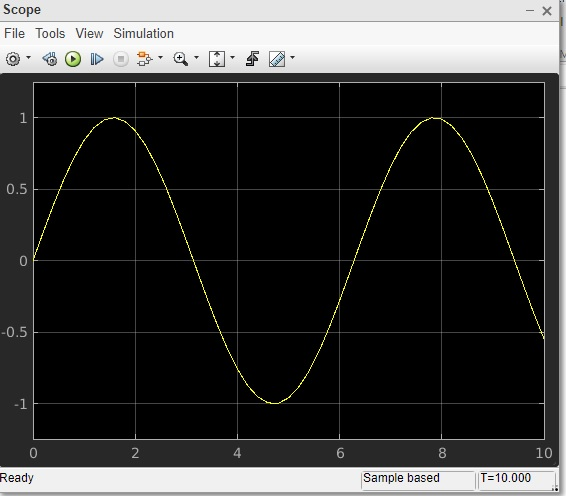# MATLAB Simulink - MATLAB Script

In this chapter, we will use MATLAB script to create a model. We do have a direct and easy method to create a model by just picking the blocks we need. But, writing the code to create a model can sometimes help you to automate a task as your projects get complex.

So, let us learn how to create models by using the Application Programming Interfaces (APIs) as discussed below.

We will create a very simple sine wave model. For that we need sine wave and scope blocks.

Inside the MATLAB command window, we can use API to create the model and blocks. To create a new model, the API is as follows −

```new_system('matlabmodel')
```

Here, matlabmodel is the name of the model. You can open the model by using open_system() with the name of the model as parameter to the function.

The command is as shown below −

```open_system('matlabmodel')
```

When you click enter, the model is opened as shown below −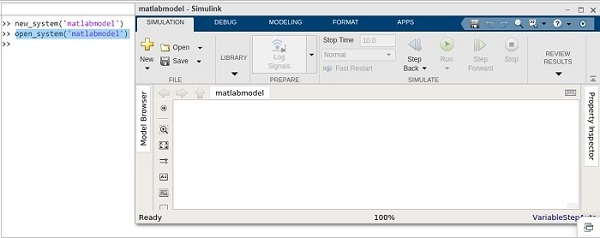Now, let us add sine wave block to it. The command is to add_block(source, dest).

You will get the source of the block from Simulink library browser.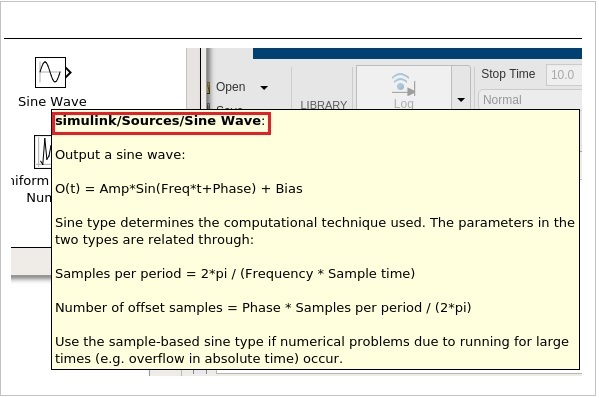The highlighted code is the source of sine wave. Let us add that in the add_block as shown below −

```add_block('simulink/Sources/Sine Wave','matlabmodel/Sine')
```

The following screen will appear on your computer −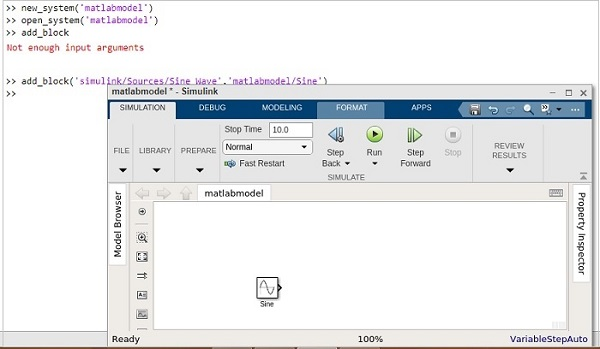Let us now add the scope block, as mentioned below −

```add_block('simulink/Sinks/Scope','matlabmodel/Scope', ‘Position’ , [200 315 135 50])
```

The model shows the scope block as shown below −You can make use of position inside the add_block to position the block properly.

For example,

```add_block(‘simulink/Sinks/Scope’, ‘matlabmodel/Scope’, ‘Position’ , [200 315 135 50]
```

Now, let us connect the line between the sine wave and scope by using the command as shown below −

```add_line('matlabmodel', 'Sine/1', 'Scope/1');
```

For add_line, you have to pass the name of your model, followed by the block name and the input port of the blocks.

So now, we want to connect the first output port of sine wave with the first input port of scope.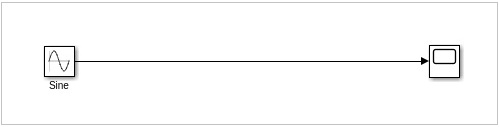Let us now run the simulation by using the command below −

```result = sim('matlabmodel');
```

Now to view the simulation result, run one more command as shown below −

```open_system('matlabmodel/Scope');
```

You will get the output inside the scope as follows −